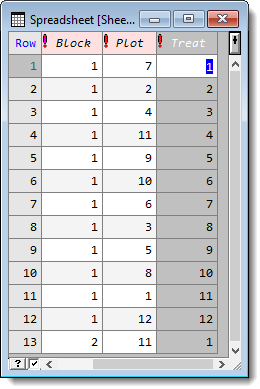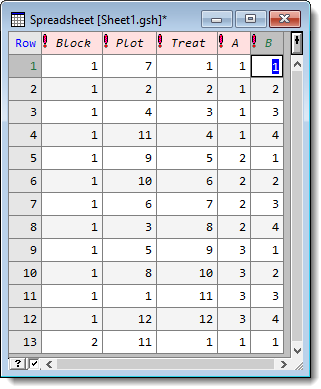1. Home
2. Dividing a Factor

# Dividing a Factor

Note: You can also combine several factors, to create a new factor with levels that represent all combinations of the combined factors, or only those present in the data.

A factor column can be divided into two or more new factors within a spreadsheet. Factor division may be useful when generating factors for a design, such as a Latin square, to study several factors and their interactions. For a 12 by 12 Latin square you may have a single factor that you want to divide into two factors A and B with 2 and 4 levels respectively. The factors A and B could be derived by dividing the 12 level treatment factor into two factors with levels (1,1,1,1,2,2,2,2,3,3,3,3) and (1,2,3,4,1,2,3,4,1,2,3,4) respectively.

The maximum number of factors that can be produced from the divide operation is six. If you want to divide a factor into more than six new factors, use this method again on the resulting factors from the previous split. For each factor that is created from the split the product of the number of levels of the resulting factors must be the same as the number of levels of the factor being divided.

The Divide Factor dialog calculates the divisors for the number of levels in the factor being divided then uses these to initialize the fields for the number of levels of each factor. If the number of levels does not have enough divisors, the dialog will not let you specify that number of resulting factors. For example if the number of levels is 12, then at most only 3 factors can be formed as 12 has prime divisors of 2, 2, and 3. If the number of levels of a factor is prime, then a warning will be provided, as this factor may not be divided.
The resulting factor columns are added back to the spreadsheet containing the original factor.

1. Put the cursor in the spreadsheet column you want to divide then select Spread | Factor | Divide.2. Select the Number of resulting factors.
If the number of levels of the factor to be divided has at most n prime divisors, then this number must be less or equal to n.3. Enter a name for each factor and edit the number of levels if required.
The Factor levels product is displayed at the bottom left of the dialog.